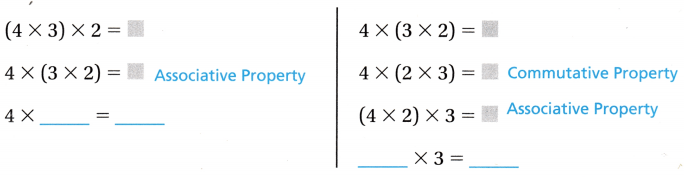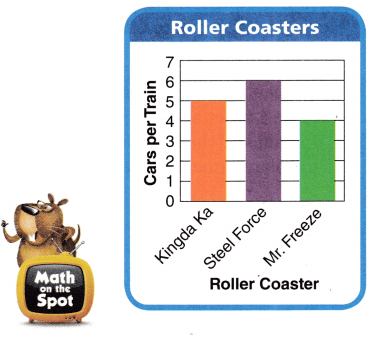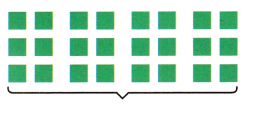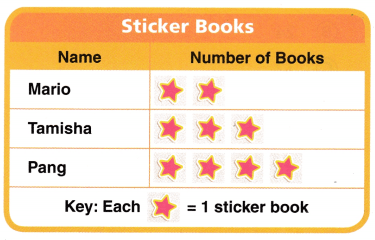# Texas Go Math Grade 3 Lesson 8.2 Answer Key Associative Property of Multiplication

Refer to our Texas Go Math Grade 3 Answer Key Pdf to score good marks in the exams. Test yourself by practicing the problems from Texas Go Math Grade 3 Lesson 8.2 Answer Key Associative Property of Multiplication.

## Texas Go Math Grade 3 Lesson 8.2 Answer Key Associative Property of Multiplication

Essential Question
How can you use the Associative Property of Multiplication to find products?

Connect You have learned the Associative Property of Addition. When the grouping of the addends is changed, the sum stays the same.
(2 + 3) + 4 = 2 + (3 + 4)

Math Idea
Always multiply the numbers inside the parentheses first.

The Associative Property of Multiplication states that when the grouping of the factors is changed, the product is the same. It is also called the Grouping Property of Multiplication.

Unlock the Problem

Each car on the roller coaster has 2 rows of seats. Each row has 2 seats. There are 3 cars in each train. How many seats are on each roller coaster train?

• Underline what you need to find.
• Describe the grouping of the seats.

Use an arrayYou can use an array to show 3 × (2 × 2).
3 × (2 × 2) = __
3 × ___ = ___
So, there are 3 cars with 4 seats in each car.
There are ___ seats on each roller coaster train.

You can change the grouping with parentheses and the product is the same.
3 × (2 × 2) = __
____ × 2 = ____

Math Talk
Mathematical Processes
Explain why the products 3 × (2 × 2) and (3 × 2) × 2 are the same.
3 × (2 × 2) and (3 × 2) × 2 When the grouping of the addends is changed, the sum stays the same.

Explanation:
The Associative Property of Multiplication states that when the grouping of the factors is changed, the product is the same. It is also called the Grouping Property of Multiplication.
When the grouping of the addends is changed, the sum stays the same.
3 × (2 × 2) = 3 × 4 = 12.
(3 × 2) × 2 = 6 × 2 = 12.

Example
Use the Commutative and Associative Properties.

You can also change the order of the factors.
The product is the same.Share and Show

Question 1.
Find the product of 5, 2, and 3. Write another way to group the factors. Is the product the same? Why?
Product is same because numbers order has changed yet not the numbers.
5 × (3 × 2)  is commutative property.
=> 5 × 6 = 30.
(5 × 2) × 3 is associative property.
=> 10 × 3 = 30.

Explanation:
Product: (5 × 2) × 3
5 × (2 × 3) is associative property.
=> 5 × 6 = 30.
5 × (3 × 2)  is commutative property.
=> 5 × 6 = 30.
(5 × 2) × 3 is associative property.
=> 10 × 3 = 30.

Write another way to group the factors. Then find the product.
Question 2.
(2 × 1) × 7
Product: 1 × (2 × 7) = 1 × 14 = 14.

Explanation:
Product:
(2 × 1) × 7 = 2 × 7 = 14.
Another way:
Change order: 1 × 2 × 7
=> 1 × (2 × 7) = 1 × 14 = 14.

Question 3.
3 × (3 × 4)
Product:
(3 × 4) × 3= 12 × 3 = 36.

Explanation:
Product:
3 × (3 × 4) = 3 × 12 = 36.
Another way:
Change order: 3 × 4 × 3
=> (3 × 4) × 3= 12 × 3 = 36.

Question 4.
5 × (2 × 5)
Product: (5 × 5) × 2 = 25 × 2 = 50.

Explanation:
Product:
5 × (2 × 5) = 5 × 10 = 50.
Another way:
Change order: 5 × 5 × 2
=> (5 × 5) × 2 = 25 × 2 = 50.

Question 5.
3 × (2 × 6)
Product: (3 × 6) × 2 = 18 × 2 = 36.

Explanation:
Product:
3 × (2 × 6) = 3 × 12 = 36.
Another way:
Change order: 3 × 6 × 2
=> (3 × 6) × 2 = 18 × 2 = 36.

H.O.T. Algebra Find the unknown factor.

Question 6.
7 × (2 × ___) = 56
7 × (2 × __4__) = 56.

Explanation:
7 × (2 × ___) = 56.
=> 7 × 2 × ?? = 56.
=> (7 × 2) × ?? = 56.
=> 14 × ?? = 56
=> ?? = 56 ÷ 14
=> ?? = 4.

Question 7.
60 = (2 × ___) × 6
60 = (2 × _5__) × 6

Explanation:
60 = (2 × ___) × 6.
=> 60 = 2 × ?? × 6
=> 60 = ?? × (2 × 6)
=> 60 = ?? × 12
=> 60 ÷ 12 = ??
=> 5 = ??

Question 8.
___ × (2 × 2) = 32
_8__ × (2 × 2) = 32.

Explanation:
___ × (2 × 2) = 32.
=> ?? × 4 = 32.
=> ?? = 32 ÷ 4
=> ?? = 8.

Question 9.
42 = 7 × (2 × __)
42 = 7 × (2 × _3_).

Explanation:
42 = 7 × (2 × __)
=> 42 = 7 × 2 × ??
=> 42 = (7 × 2) × ??
=> 42 = 14 × ??
=> 42 ÷ 14 = ??
=> 3 = ??.

Question 10.
8 × (5 × ___) = 4o
8 × (5 × _1__) = 40.

Explanation:
8 × (5 × ___) = 40.
=> 8 × 5 × ?? = 40.
=> ( 8 × 5) × ?? = 40.
=> 40 × ?? = 40.
=> ?? = 40 ÷ 40
=> ?? = 1.

Question 11.
0 = ____ × (25 × 1)
0 = __0__ × (25 × 1)

Explanation:
0 = ____ × (25 × 1)
=> 0 = ?? × (25 × 1)
=> 0 = ?? × 25
=> 0 ÷ 25 = ??
=> 0 = ??

Problem Solving
Use the graph for 12-13.
Question 12.
Multi-Step Each car on the Steel Force train has 3 rows with 2 seats in each row. How many seats are on the train? Draw a quick picture.Number of seats are on the train = 6.

Explanation:
Number of rows each car on the Steel Force train has = 3.
Number of seats in each row each car on the Steel Force train has = 2.
Number of seats are on the train = Number of rows each car on the Steel Force train has × Number of seats in each row each car on the Steel Force train has
= 3 × 2
= 6.Question 13.
H.O.T. Multi-Step A Kingda Ka train has 4 seats per car, but the last car has only 2 seats. How many seats are on one Kingda Ka train?
Number of seats are on one Kingda Ka train = 18.

Explanation:
Number of seats per car a Kingda Ka train has = 4.
Number of seats last car a Kingda Ka train has = 2.
Number of cars a Kingda Ka train has = 5.
Number of seats are on one Kingda Ka train = (Number of cars a Kingda Ka train has × Number of seats per car a Kingda Ka train has) – Number of seats last car a Kingda Ka train has
= (5 × 4) – 2
= 20 – 2
= 18.

Question 14.
Sense or Nonsense? Each week, Kelly works 2 days for 4 hours each day and earns $5 an hour. Len works 5 days for 2 hours each day and earns$4 an hour. Kelly says they both earn the same amount. Does this statement make sense? Explain.
Yes, the statement makes sense because both earn same amount of $40. Explanation: Number of days Kelly works = 2. Number of hours each day Kelly works = 4. Cost of amount for an hour =$5.
Amount of money Kelly works a week = Number of days Kelly works × (Number of hours each day Kelly works × Cost of amount for an hour )
= 2 × (4 × $5) = 2 ×$20
= $40. Number of days Len works = 5. Number of hours each day Len works = 2. Cost of amount for an hour =$4.
Amount of money Len works a week = Number of days Len works × (Number of hours each day Len works × Cost of amount for an hour )
= 5 × (2 × $4) = 5 ×$8
= $40. Question 15. Representations What number sentence does this array represent? Write another way to group the factors.Answer: Another way: Number of columns × Number of rows = 8 × 3 = 24. Explanation: Number of rows = 3. Number of columns = 8. Array represent = Number of rows × Number of columns = 3 × 8 = 24. Another way: Number of columns = 8. Number of rows = 3. Array represent = Number of columns × Number of rows = 8 × 3 = 24. Daily Assessment Task Fill in the bubble for the correct answer choice. You can use an array, area model, or a strip diagram to represent and solve. Question 16. Michael plays a fishing game at the school carnival. He plays 4 times and catches 3 fish each time. For each fish Michael catches, he wins a set of 3 toy planes. How many toy planes does Michael win? (A) 18 (B) 12 (C) 36 (D) 9 Answer: Number of planes Michael wins = 36. (C) 36. Explanation: Number of times he plays = 4. Number of fishes he catches each time = 3. Number of set of toy planes when he wins = 3. Number of planes Michael wins = Number of times he plays × ( Number of fishes he catches each time × Number of set of toy planes when he wins ) = 4 (3 × 3) = 4 × 9 = 36. Question 17. Emma helps at a farm 2 days each week. She works 4 hours each day and earns$8 for each hour. How much money does Emma earn each week?
(A) $64 (B)$40
(C) $48 (D)$32
Amount of money  Emma earns each week = $64. (A)$64.

Explanation:
Number of days each week Emma helps at a farm = 2.
Number of hours each day she works = 4.
Cost of amount she earns each hour = $8. Amount of money Emma earns each week = Number of days each week Emma helps at a farm × (Number of hours each day she works × Cost of amount she earns each hour) = 2 × (4 ×$8)
= 2 × 32
= $64. Question 18. Multi-Step Mrs. Lee has 25 baskets of books. She wants to put them on the 2 bookcases in her classroom. Each bookcase has 4 shelves. Each shelf can hold 3 baskets. How many baskets will be left over? (A) 7 (B) 1 (C) 10 (D) 5 Answer: Number of baskets will be left over = 1. (B) 1. Explanation: Number of baskets of books Mrs. Lee has = 25. Number of bookcases in her classroom she wants to put them = 2. Number of shelves each bookcase has = 4. Number of baskets each shelf can hold = 3. Number of baskets will be left over = Number of baskets of books Mrs. Lee has -[ Number of bookcases in her classroom she wants to put them × (Number of shelves each bookcase has × Number of baskets each shelf can hold)] = 25 – [2 × (4 × 3)] = 25 – (2 × 12) = 25 – 24 = 1. Texas Test Prep Question 19. Mark has 2 rows of 3 car models on each of his 3 shelves. How many car models does he have? (A) 11 (B) 9 (C) 8 (D) 18 Answer: Number of car models he have = 18. (D) 18. Explanation: Number of rows Mark has = 2. Number of car models Mark has = 3. Number of cars on each shelves = 3. Number of car models he have = Number of rows Mark has × (Number of car models Mark has × Number of cars on each shelves) = 2 × (3 ×3) = 2 × 9 = 18. ### Texas Go Math Grade 3 Lesson 8.2 Homework and Practice Answer Key Write another way to group the factors. Then find the product. Question 1. (3 × 2) × 5 Answer: (3 × 2) × 5 = 3 × (2 × 5) = 30. Explanation: Another way: (3 × 2) × 5 = 3 × (2 × 5) = 3 × 10 = 30. Question 2. 6 × (2 × 2) Answer: 6 × (2 × 2) = (6 × 2) × 2 = 24. Explanation: Another way: 6 × (2 × 2) = (6 × 2) × 2 = 12 × 2 = 24. Question 3. 5 × (2 × 4) Answer: 5 × (2 × 4) = (5 × 2) × 4 = 40. Explanation: Another way: 5 × (2 × 4) = (5 × 2) × 4 = 10 × 4 = 40. Question 4. (2 × 3) × 6 Answer: (2 × 3) × 6 = 2 × (3 × 6) = 36. Explanation: Another way: (2 × 3) × 6 = 2 × (3 × 6) = 2 × 18 = 36. Problem Solving Use the pictograph for 5-7. Question 5. Mario’s sticker books have 4 rows of 3 stickers in each row. How many stickers are in Mario’s books? Draw a picture.Answer: Number of stickers are in Mario’s books = 12. Explanation: Number of rows Mario’s sticker books = 4. Number of stickers in each row = 3. Number of stickers are in Mario’s books = Number of rows Mario’s sticker books × Number of stickers in each row = 4 × 3 = 12. Question 6. Pang’s sticker books have 3 rows of 3 stickers. He uses 5 stickers. How many stickers are still in the sticker books? Answer: Number of stickers are still in the sticker books = 4. Explanation: Number of rows Pang’s sticker books = 3. Number of stickers in each row = 3. Number of stickers used by Pang’s = 5. Number of stickers are still in the sticker books = (Number of rows Pang’s sticker books × Number of stickers in each row) – Number of stickers used by Pang’s = (3 × 3) – 5 = 9 – 5 = 4. Question 7. Tamisha’s sticker books have 3 rows of 3 stickers in each row. If Mario’s sticker books have 4 rows of 3 stickers in each row, who has more stickers? Explain. Answer: Mario’s sticker books has 3 more stickers than Tamisha’s sticker books. Explanation: Number of rows Tamisha’s sticker books = 3. Number of stickers in each row = 3. Number of stickers are in Tamisha’s books = Number of rows Tamisha’s sticker books × Number of stickers in each row = 3 × 3 = 9. Number of rows Mario’s sticker books = 4. Number of stickers in each row = 3. Number of stickers are in Mario’s books = Number of rows Mario’s sticker books × Number of stickers in each row = 4 × 3 = 12. Difference: Number of stickers are in Mario’s books – Number of stickers are in Tamisha’s books = 12 – 9 = 3. Lesson Check Question 8. Malcolm put stamps on 5 pages of an album. He puts 3 rows of 2 stamps on each page. How many stamps does Malcolm have in his album? (A) 10 (B) 30 (C) 20 (D) 25 Answer: Number of stamps Malcolm have in his album = 30. (B) 30. Explanation: Number of pages of an album Malcolm put stamps on = 5. Number of rows he puts = 3. Number of stamps on each page he puts = 2. Number of stamps Malcolm have in his album = Number of pages of an album Malcolm put stamps on × (Number of rows he puts × Number of stamps on each page he puts) = 5 × (3 × 2) = 5 × 6 = 30. Question 9. Ana works at the library 3 days each week. She works for 2 hours each day and earns$7 each hour. How much money does Ana earn each week?
(A) $42 (B)$21
(C) $12 (D)$35
Amount of money does Ana earn each week = $42. (A)$42.

Explanation:
Number of days in a week Ana works at the library = 3.
Number of hours each day she works = 2.
Money she earns each hour = $7. Amount of money does Ana earn each week = Number of days in a week Ana works at the library × (Number of hours each day she works × Money she earns each hour) = 3 × (2 ×$7)
= 3 × $14 =$42.

Question 10.
Zeff, Maggie, and Jani each make 2 kites. Each kite has 4 tails. How many tails are there on all of the kites?
(A) 8
(B) 24
(C) 12
(D) 18
Number of tails are there on all of the kites = 24.
(B) 24.

Explanation:
Number of kites each make = 2.
Number of people making kites = 3.(Zeff, Maggie, and Jani)
Number of tails each kite has = 4.
Number of tails are there on all of the kites = (Number of kites each make × Number of people making kites) × Number of tails each kite has
= (2 × 3) × 4
= 6 × 4
= 24.

Question 11.
There are 6 boxes of paints. Each box has 3 rows of 2 paint cans. Which shows how many paint cans in all?
(A) 6 + (3 × 2) = 12
(B) (6 × 3) + 2 = 20
(C) (6 × 3) × 2 = 18
(D) 6 × (3 × 2) = 36
Number of paint cans in all = 36.
(D) 6 × (3 × 2) = 36

Explanation:
Number of boxes of paints = 6.
Number of rows in each box has = 3.
Number of paints cans = 2.
Number of paint cans in all = Number of boxes of paints × (Number of rows in each box has × Number of paints cans)
= 6 × (3 × 2)
= 6 × 6
= 36.

Question 12.
Multi-Step Gwen has 30 action figures. She puts them in 2 boxes. Each box holds 3 rows of 4 action figures. How many action figures will be left over?
(A) 24
(B) 6
(C) 8
(D) 21
Number of action figures will be left over = 6.
(B) 6.

Explanation:
Number of action figures Gwen has = 30.
Number of boxes she puts them = 2.
Number of rows each box holds = 3.
Number of action figures = 4.
Number of action figures will be left over = Number of action figures Gwen has – [Number of boxes she puts them × (Number of rows each box holds × Number of action figures)]
= 30 – [2 × (3 × 4)]
= 30 – [2 × 12]
= 30 – 24
= 6.

Question 13.
Multi-Step Reggie has 65 quilt patches. He makes 2 quilts with 5 rows of 6 patches in each quilt. How many quilt patches will be left over?
(A) 43
(B) 8
(C) 5
(D) 52
Number of quilt patches will be left over = 5.
(C) 5.

Explanation:
Number of quilt patches Reggie has = 65.
Number of quilts he makes = 2.
Number of rows he makes = 5.
Number of patches in each quilt = 6.
Number of quilt patches will be left over = Number of quilt patches Reggie has – [ Number of quilts he makes × (Number of rows he makes × Number of patches in each quilt )]
= 65 – [2 × (5 × 6)]
= 65 – [2 × 30]
= 65 – 60
= 5.

Scroll to Top